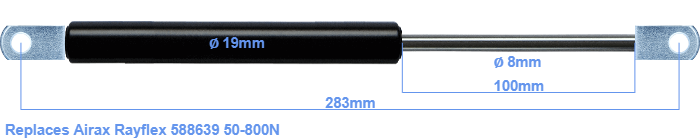# Replacement for Airax Rayflex 588639 50-800N

\$30.81

Replacement gas spring for the Airax Rayflex 588639 50-800 Newton. The eye has a thickness of 10mm and a hole diameter of 8.1mm. Brand: Stabilus Industry Line.

Please note:
• The thickness of the eye of this replacement is 10mm instead of 5mm.
• The diameter of the tube of this replacement gas spring is 19mm instead of 20mm.
•  Force Choose an option50 Newton60 Newton80 Newton100 Newton120 Newton140 Newton150 Newton160 Newton180 Newton200 Newton220 Newton240 Newton250 Newton260 Newton280 Newton300 Newton320 Newton340 Newton350 Newton360 Newton380 Newton400 Newton420 Newton440 Newton450 Newton460 Newton480 Newton500 Newton520 Newton540 Newton550 Newton560 Newton580 Newton600 Newton620 Newton640 Newton650 Newton660 Newton680 Newton700 Newton720 Newton740 Newton750 Newton760 Newton780 Newton800 NewtonClear
This gas spring is also known as 5000588639, 588639.00.The diameter of the cylinder of this gas spring is 19 millimeters. The rod has a diameter of 8 mm. The stroke (the retracting part) has a length of 100 mm. The total length of this replacement is exactly 283 mm. Keep in mind that this is the length between the centers of the mounting parts. The distance from thread to thread (without mounting parts) is 245 mm. The force of this replacement is 50-800 Newton. This is not an original gas spring produced by Airax Rayflex, but it can replace a Airax Rayflex gas spring. This a gas spring with the brand Stabilus Industry Line. Nevertheless, this gas spring can be used as replacement gas spring, because the dimensions and forces are almost equal.
Category: